# Multiplying Proper Fractions

Multiplying Proper Fractions• Finding   1 / 2   of   1 / 3   is the same as finding   1 / 2   ×   1 / 3  .
•   1 / 3   means to divide the shape into 3 equal parts.
• We now find one half of this by dividing it into two equal parts.
• We now have 1 part out of 6 equal parts.
•   1 / 2   ×  1 / 3   =   1 / 6  .
• We can simply multiply the of the fractions to get the numerator of the answer.
• We can simply multiply the of the fractions to get the denominator of the answer.
• 1 × 1 = 1, which goes on top.
• 2 × 3 = 6, which goes on the bottom.

Multiply the numerators on the top to get the numerator of the answer.

Multiply the denominators on the bottom to get the denominator of the answer.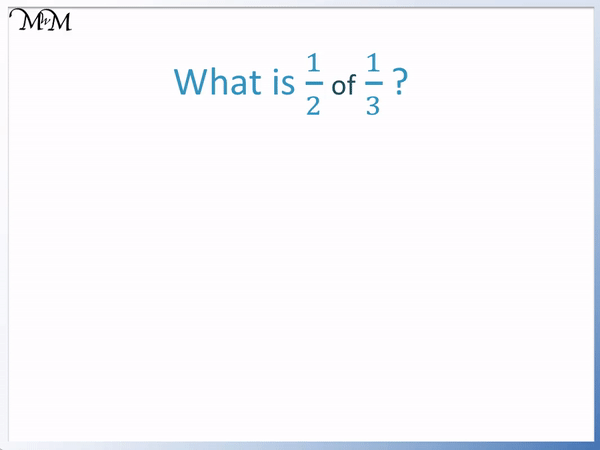• We have   1 / 2   ×   1 / 3  .
• Multiply the numbers on the tops of the fractions (the numerators) together.
• 1 × 1 = 1 and so, 1 is the numerator on the top of the answer.
• Multiply the numbers on the bottoms of the fractions (the denominators) together.
• 2 × 3 = 6 and so, 6 is the denominator on the bottom of the answer.
•   1 / 2   ×   1 / 3   =   1 / 6  .Supporting Lessons# How to Multiply Proper Fractions

## How to Multiply Proper Fractions

A proper fraction is a fraction that has a smaller number on top (as the numerator) and larger number on the bottom (as the denominator).

To multiply proper fractions use the following steps:

1. Multiply the numerators on the top of the fractions.
2. This result will be the numerator on the top of the answer.
3. Multiply the denominators on the bottom of the fractions.
4. This result will be the denominator on the bottom of the answer.

Here is an example of multiplying the fractions of one half by one third.We are asked, “What is   1 / 2   ×   1 / 3  ?”

The numerators are the numbers on the tops of the fractions. We multiply the numerator of the first fraction by the numerator of the second fraction to get the numerator on the top of our answer.

1 × 1 = 1

The denominators are the numbers on the bottoms of the fractions. We multiply the denominator of the first fraction by the denominator of the second fraction to get the denominator on the bottom of our answer.

2 × 3 = 6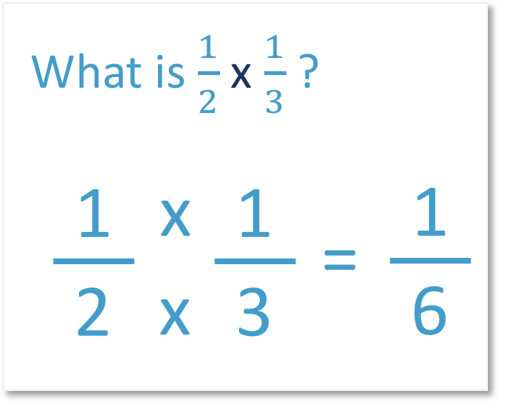1 / 2   ×   1 / 3   =   1 / 6  .Here is another example of multiplying proper fractions using the steps above.

We have   1 / 4   ×   1 / 3  .

First, we multiply the numerators.

1 × 1 = 1

So 1 is the numerator on top of our answer.

Next we multiply the denominators.

4 × 3 = 12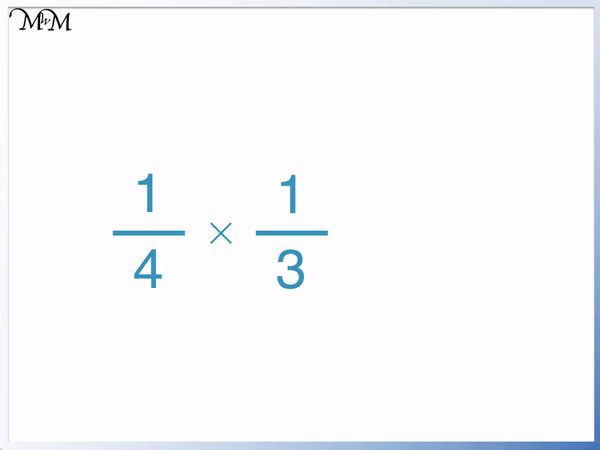1 / 4   ×   1 / 3   =   1 / 12  .

In this example of multiplying proper fractions we have   2 / 5   ×   2 / 3  .

We start by multiplying the numerators on the tops of the two fractions.

2 × 2 = 4

4 is the numerator on top of our answer.

Next we multiply the two denominators on the bottoms of the fractions together.

5 × 3 = 15

15 is the denominator on the bottom of our answer.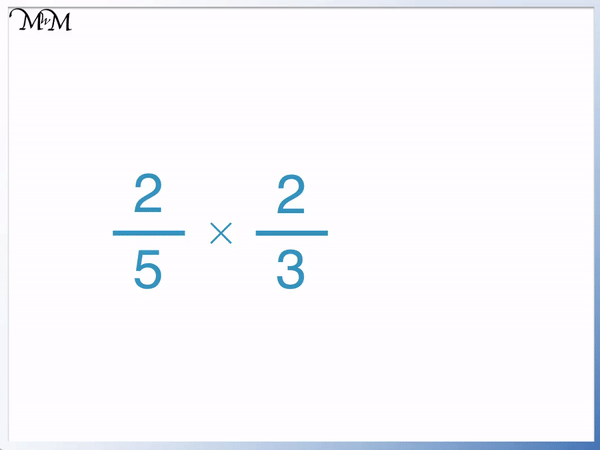2 / 5   ×   2 / 3   =   4 / 15  .

In this example we are multiplying the proper fractions of   1 / 5   ×   3 / 4  .

Multiplying the numerators we have:

1 × 3 = 3

Multiplying the denominators we have:

5 × 4 = 201 / 5   ×   3 / 4   =   3 / 20  .

## Why do Proper Fractions get Smaller when you Multiply them?

Multiplying a fraction by a proper fraction always makes it smaller.

This is because a proper fraction has a smaller number on top as its numerator compared to a larger number on the bottom as its denominator. When we multiply by a fraction we are multiplying by the number on top but dividing by the number on the bottom.

So we are multiplying by a smaller number than we are dividing by. This means that our answer will be smaller than we started with.

In this example we will start with the fraction   1 / 3  .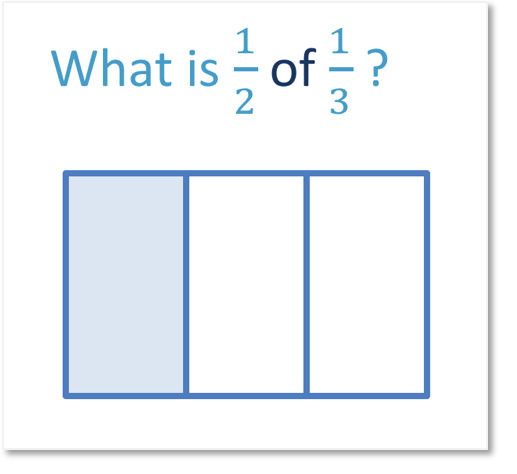We will find   1 / 2   of   1 / 3  .

This means that we can draw a line through the fraction of   1 / 3  , splitting it in half.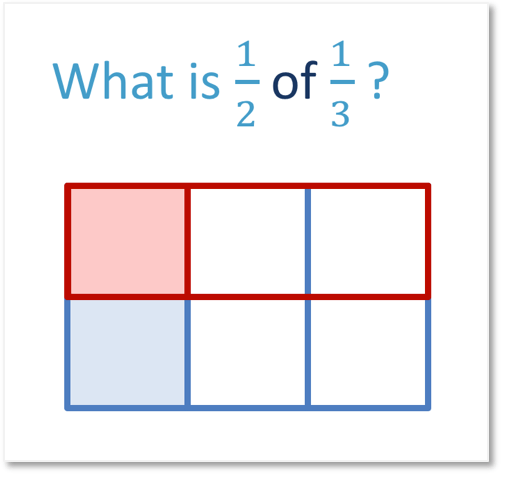We now have 6 parts in total rather than 3.

We have 1 part shaded out of 6 parts in total.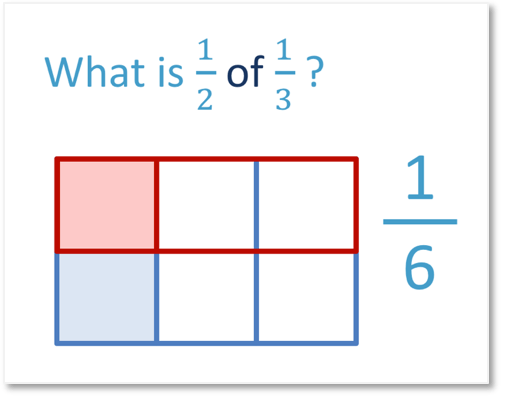We can see that multiplying one third by one half made it smaller because it split the fraction in half.

We found a fraction of a fraction.

In Maths the word ‘of’ can be replaced with a multiplication.Now try our lesson on Simplifying Fractions before Multiplying where we learn how to multiply larger fractions by simplifying them first.error: Content is protected !!